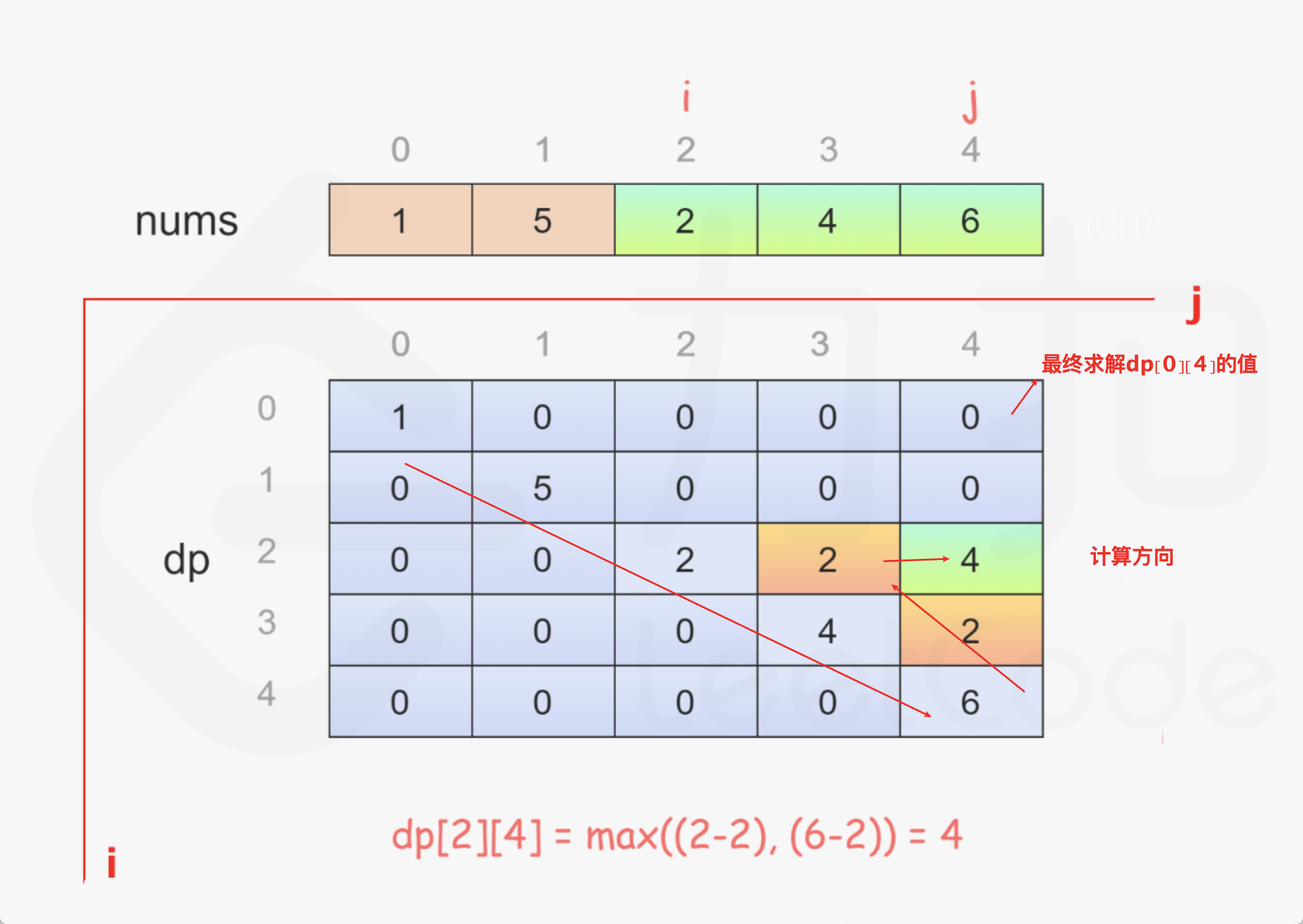# 486. 预测赢家

``````class Solution {

public boolean PredictTheWinner(int[] nums) {
int length = nums.length;
//偶数长度下先手玩家必赢
if (length % 2 == 0) {
return true;
}
//进行动态规划求解
int[][] dp = new int[length][length];
for (int i = 0; i < length; i++) {
dp[i][i] = nums[i];
}
for (int i = length - 2; i >= 0; i--) {
for (int j = i+1; j < length; j++) {
dp[i][j] = Math.max(nums[i] - dp[i + 1][j], nums[j] - dp[i][j - 1]);
}
}
return dp[length - 1] >= 0;
}

}
```````dp[i][j]`的值代表，在i-j这个范围内的数字，我（先手）和对手经过各自的最优选择之后，我能领先的分数。注意这里的先手不考虑全局的范围，就考虑在i-j的范围内。也可以理解为，把原先的大数组，拆成多个i-j的小数组，每个小数组的情况都去计算结果，提前将结果缓存下来，避免使用递归方法调用时带来的重复计算。

`i=j`的时候，即只有一个数的情况下，那么先手玩家只能选择这个数，后手玩家没得选。所以`dp[i][j]=dp[i][i]=nums[i]`，由此我们可以计算出表格中对角线的部分。

`i<j`的时候，先手玩家只有两种选择，选择`nums[i]`或者`nums[j]`，当选择`nums[i]`时，先手玩家可以领先分数为（当前选择的分数减去剩下的数组中最优解下能领先的分数）：`nums[i]-dp[i+1][j]`，当选择`nums[j]`时，先手玩家可以领先分数为：`nums[j]-dp[i][j-1]`。此时先手玩家选择i还是j位置的数字取决于上述计算的2个分数哪个大，所以可以得出`dp[i][j]=max(nums[i]-dp[i+1][j],nums[j]-dp[i][j-1])`。这个公式比较难理解，也是重点所在，可以多理解一会。